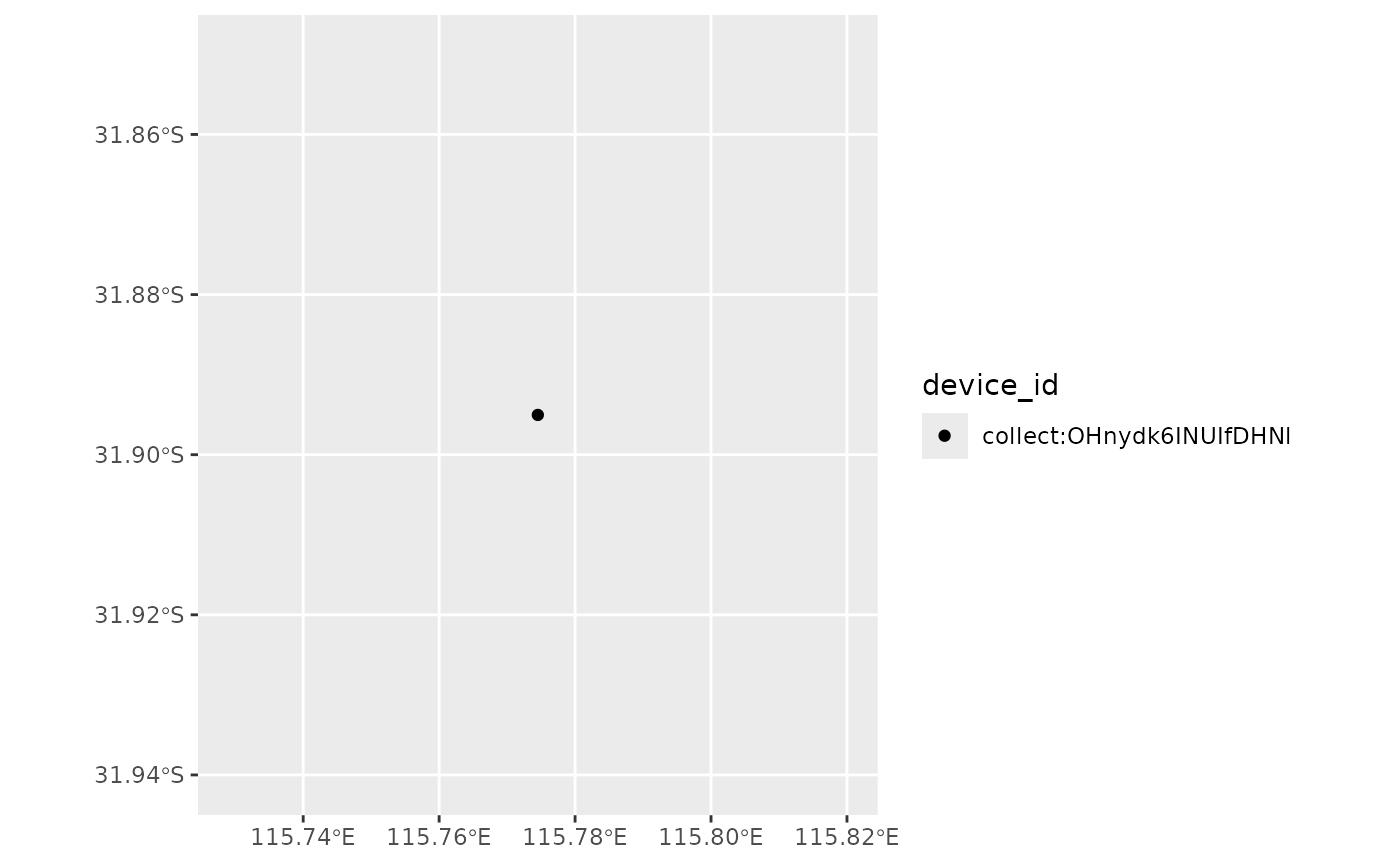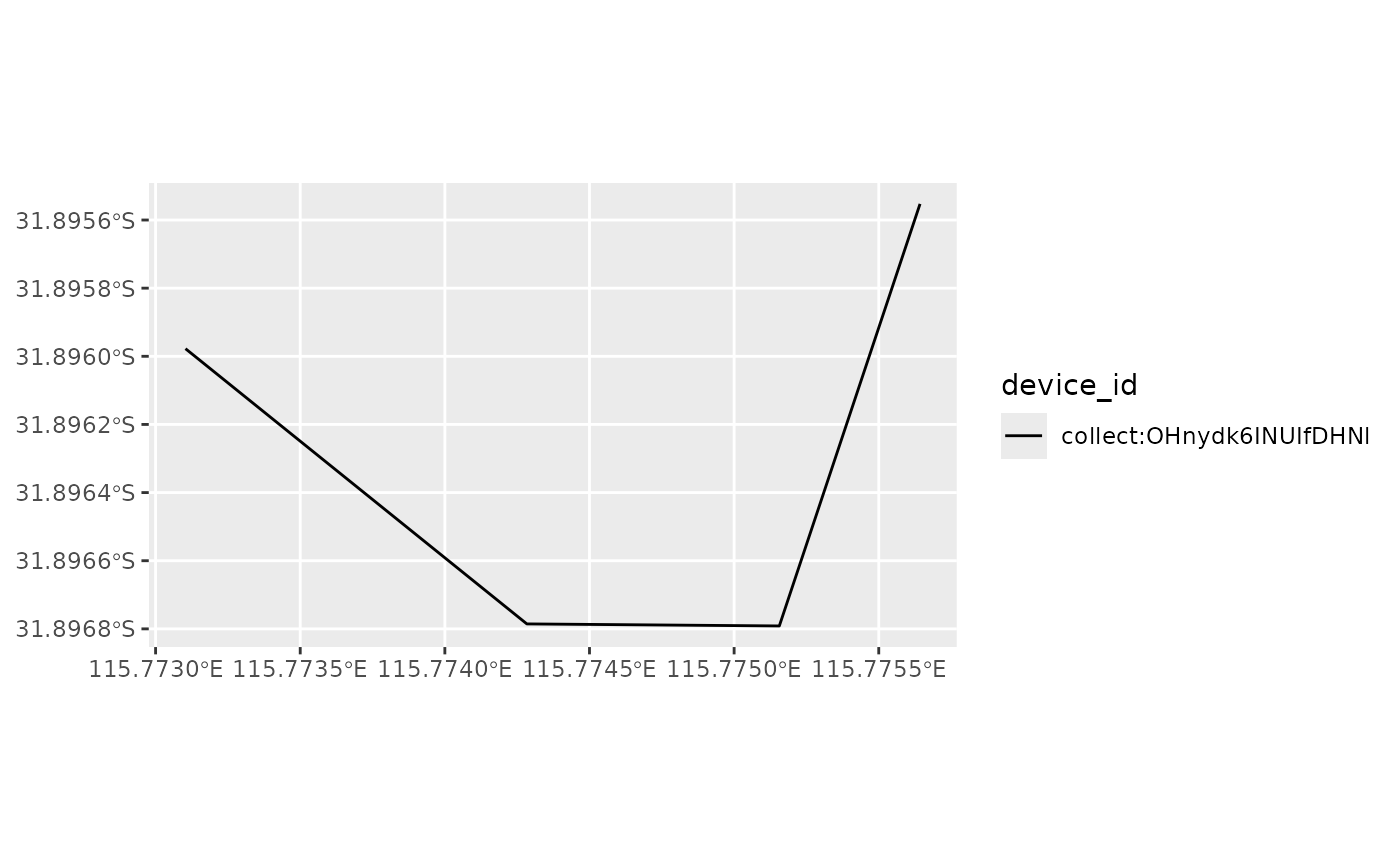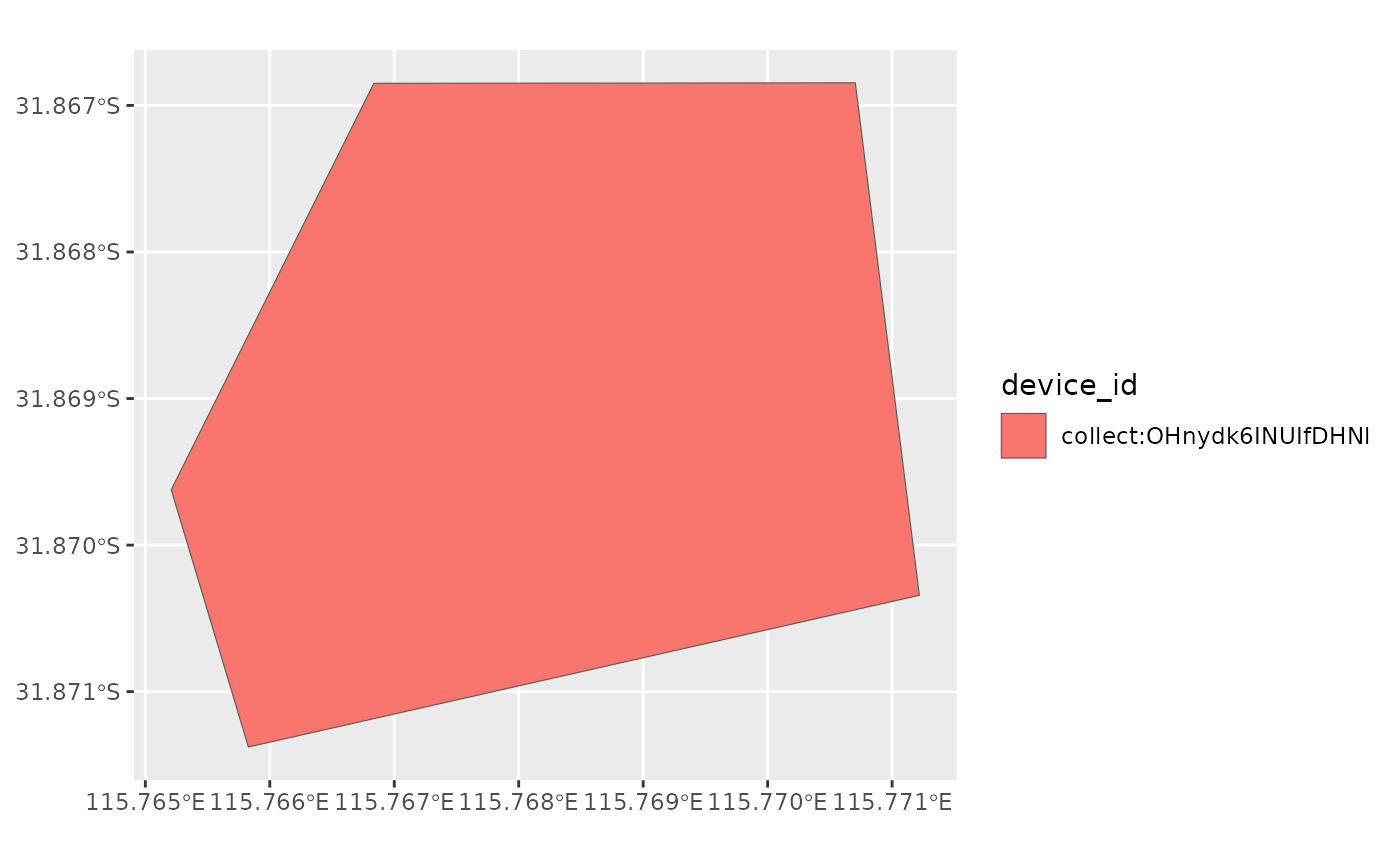For forms with spatial types, such as geopoint, geotrace, or geoshape, `ruODK` gives two options to access the captured spatial data.

Firstly, to make spatial data as simple and accessible as possible, `ruODK` extracts the lat/lon/alt/acc from geopoints, as well as from the first coordinate of geotraces and geoshapes into separate columns. This works for both GeoJSON and WKT. The extracted columns are named as the original geofield appended with `_latitude`, `_longitude`, `_altitude`, and `_accuracy`, respectively.

Secondly, this vignette demonstrates how to turn the spatial data types returned from ODK Central into spatially enabled objects. To do so, we have to address two challenges.

The first challenge is to select which of the potentially many spatial fields which an ODK form can capture shall be used as the primary geometry of a native spatial object, such as an `sf` SimpleFeature class. If several spatial fields are captured, it is up to the user to choose which field to use as primary geometry.

The second challenge is that the parsed data from ODK Central is a plain table (`tbl_df`) in which some columns contain spatial data. Well Know Text (WKT) is parsed as text columns, whereas GeoJSON (nested JSON) is parsed as list columns.

Most spatial packages require either atomic coordinates in separate columns, which works well for points (latitude, longitude, altitude), or the data to be spatially enabled. This vignette shows how to transform a `tbl_df` with a column containing (point, line, or polygon) WKT into a spatially enabled `sf` object.

``````library(ruODK)

# Visualisation
library(leaflet)
library(ggplot2)
library(lattice)
# library(tmap) # Suggested but not included here yet

# Spatial
can_run <- require(sf) && require(leafem) && require(mapview) && require(terra)
# Fix https://github.com/r-spatial/mapview/issues/313
# option 'fgb' requires GDAL >= 3.1.0
if (require(mapview)) {
mapview::mapviewOptions(
fgb = FALSE,
basemaps = c(
"Esri.WorldImagery",
"OpenTopoMap",
"OpenStreetMap"
),
layers.control.pos = "topright"
)
}``````

### Data

The original data shown in this vignette are hosted on a ODK Central server which is used for the `ruODK` package tests. The form we show here contains every spatial widget supported by ODK Build for every supported spatial field type.

With working credentials to the ODK Central test server we could download the data directly.

``````# Set ruODK defaults to an ODK Central form, choose tz and verbosity
ruODK::ru_setup(
url = get_test_url(),
pid = get_test_pid(),
fid = get_test_fid_wkt(),
un = get_test_un(),
pw = get_test_pw(),
odkc_version = 0.8,
tz = "Australia/Perth",
verbose = TRUE
)
data_wkt <- ruODK::odata_submission_get(wkt = TRUE)
data_gj <- ruODK::odata_submission_get(wkt = FALSE)``````

To allow users to build this vignette without credentials to the ODK Central test server, `ruODK` provides above form data also as package data.

``````data("geo_wkt", package = "ruODK")
data("geo_gj", package = "ruODK")``````

### Map geopoints

We can turn data with a text column containing WKT into an `sf` (SimpleFeatures) object.

In addition, we can leave the `tbl_df` as non-spatial object, and instead use the separately extracted latitude, longitude, altitude, and accuracy individually e.g. to plot a Leaflet map.

``````geo_sf_point <- geo_wkt %>% sf::st_as_sf(wkt = "point_location_point_gps")
# ODK Collect captures WGS84 (EPSG:4326)
sf::st_crs(geo_sf_point) <- 4326``````

#### Mapview using sf

``mapview::mapview(geo_sf_point, col.regions = sf::sf.colors(10))``

#### GGplot using sf

``````ggplot2::ggplot() +
ggplot2::geom_sf(data = geo_sf_point, ggplot2::aes(fill = device_id))``````#### Leaflet using sf

``````leaflet::leaflet(data = geo_sf_point) %>%
leaflet::addMarkers(label = ~device_id, popup = ~device_id)``````

#### Leaflet using extracted coordinate components in tbl_df

``````leaflet::leaflet(data = geo_wkt) %>%
lng = ~point_location_point_gps_longitude,
lat = ~point_location_point_gps_latitude,
label = ~device_id,
popup = ~device_id
)``````

### Map geotraces (lines)

We use `sf::st_as_sf` on a text column containing a WKT geotrace.

``````geo_sf_line <- geo_wkt %>% sf::st_as_sf(wkt = "path_location_path_gps")
# ODK Collect captures WGS84 (EPSG:4326)
sf::st_crs(geo_sf_line) <- 4326``````

#### Mapview using sf

``mapview::mapview(geo_sf_line, col.regions = sf::sf.colors(10))``

#### GGplot using sf

``````ggplot2::ggplot() +
ggplot2::geom_sf(data = geo_sf_line, ggplot2::aes(fill = device_id))``````#### Leaflet using sf and extracted coordinates

You can show either first extracted coordinate components from plain `tbl_df` or show the full polygons using leafem. See the mapview article on extra functionality.

``````leaflet::leaflet(data = geo_wkt) %>%
lng = ~path_location_path_gps_longitude,
lat = ~path_location_path_gps_latitude,
label = ~device_id,
popup = ~device_id
) %>%
leafem::addFeatures(geo_sf_line, label = ~device_id, popup = ~device_id)``````

### Map geoshapes (polygons)

Again, we’ll use `sf::st_as_sf` but select a WKT geoshape column.

``````geo_sf_poly <- geo_wkt %>% sf::st_as_sf(wkt = "shape_location_shape_gps")
# ODK Collect captures WGS84 (EPSG:4326)
sf::st_crs(geo_sf_poly) <- 4326``````

#### Mapview using sf

``mapview::mapview(geo_sf_poly, col.regions = sf::sf.colors(10))``

#### GGplot using sf

``````ggplot2::ggplot() +
ggplot2::geom_sf(data = geo_sf_poly, ggplot2::aes(fill = device_id))``````#### Leaflet using sf and extracted coordinates

You can show either first extracted coordinate components from plain `tbl_df` or show the full polygons using leafem. See the mapview article on extra functionality.

``````leaflet::leaflet(data = geo_wkt) %>%
lng = ~shape_location_shape_gps_longitude,
lat = ~shape_location_shape_gps_latitude,
label = ~device_id,
popup = ~device_id
) %>%
leafem::addFeatures(geo_sf_poly, label = ~device_id, popup = ~device_id)``````

## Outlook

The above examples show how to turn spatial data into an `sf` object, and give very rudimentary visualisation examples to bridge the gap between spatial data coming from ODK and creating maps and further spatial analyses in R.

See the sf homepage for more context and examples. The sf cheatsheet deserves a spatial mention.

Review the options for mapview popups and the whole mapview homepage for a comprehensive overview of mapview.

The powerful visualisation package `tmap` supports `sf` objects and produces both printable and static maps as well as interactive `leaflet` maps. See the vignette “Get started”.

There are several other good entry points for all things R and spatial, including but not limited to:

The above list of examples and resources is far from comprehensive. Feel free to contribute or suggest other working examples for turning data from `ruODK` into spatial formats.